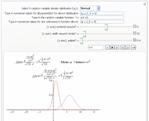UP

Simulation of distributions using Mathematica 6

By Nasser Abbasi

Last updated 10/10/09

This page contains number of Mathematica notebooks to illustrate the use of its Statistics functions. The table below contains the notebooks, the player version of the notebook (which could be played using Mathematica free player) to download the free player to play these notebooks, go to http://www.wolfram.com/solutions/interactivedeployment/publish/

Once you install the player, then you can double click on any of these (.nbp) files below (the files in the links located in the second column of the table below) and run them.

There is a simulator for discrete and continuous distributions, and one for bivariate normal distributions. Screen shots are the bottom of this page.

 Date updated Description Notebook (nb) Player version of the notebook (nbp) HTML PDF of the notebook Screen shots Discrete Distributions simulator (Using Dynamics) 1/3/2008 EXPIERMENTAL. Same as above, but the UI was designed using Mathematica Dynamics calls instead of Manipulate. Only 2 distributions.continuous Distributions simulator (Using Manipulate) December 2007 This one is for  continuous Distributions. I am working on a new version but not yet completed.Bivariate Normal simulator December 2007 Allows one to see the PDF and CDF of a bivariate normal distribution.Function of random variable December 2007 This is not completed work. Buggy code. User can enter a function (say Y=a X+b) where X is random variable of some distribution, and adjust parameters of X and see Y as a result.Small note on using Mathematica to study statistics December 2007 Showing very basic stuff.

Other Screen shots (might be from earlier versions)

 BernoulliBinomialGeometricnegativeBinomialHypergeometricPoissonExponential DensityGamma DensityNormal DistributionBeta Density